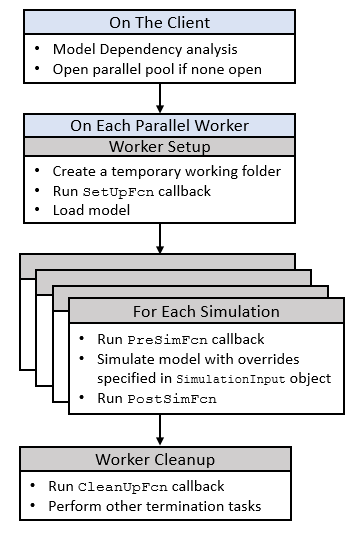Documentation

## Run Parallel Simulations

The `parsim` command allows you to run parallel (simultaneous) Simulink® simulations of your model (design). In this context, parallel runs mean multiple simulations at the same time on different workers. `parsim` makes it easy for you to run the same model with different inputs or different parameter settings in scenarios such as Monte Carlo analyses, parameter sweeps, model testing, experiment design, and model optimization. Running a single simulation in parallel by decomposing the model into smaller components and running those individual pieces simultaneously on multiple workers is currently not supported.

To run the simulations in parallel with `parsim`, you need a Parallel Computing Toolbox™ for local workers. In addition, you can use MATLAB® Parallel Server™ for multiple computer clusters, clouds, and grids. In the absence of Parallel Computing Toolbox and MATLAB Parallel Server, `parsim` runs the simulations in serial. For more information, see Parallel Computing Toolbox and MATLAB Parallel Server.

If no parallel pool exists, `parsim` creates a pool from the default cluster profile . To use a cluster other than the default, create a pool with that cluster profile before calling `parsim`.

This example runs multiple simulations in parallel for a set of sweep parameters.

```% 1) Load model model = 'sldemo_suspn_3dof'; load_system(model); % 2) Set up the sweep parameters Cf_sweep = 2500*(0.05:0.1:0.95); numSims = numel(Cf_sweep); % 3) Create an array of SimulationInput objects and specify the sweep value for each simulation simIn(1:numSims) = Simulink.SimulationInput(model); for idx = 1:numSims simIn(idx) = simIn(idx).setBlockParameter([model '/Road-Suspension Interaction'], 'Cf', num2str(Cf_sweep(idx))) end % 4) Simulate the model simOut = parsim(simIn) ```

### How `parsim` works

`parsim` runs simulations with different parameters and values based on the `Simulink.SimulationInput` object. Each `SimulationInput` object specifies one simulation of the model. An array of these objects can be created for multiple simulations. For more information, see Run Multiple Simulations.

You can use the following methods and properties on `Simulink.SimulationInput` object:

• `setVariables` - Change variables in base workspace, data dictionary, or model workspace

• `setBlockParameters` - Change block parameters

• `setModelParameters` - Change model parameters

• `setPreSimFcn` - Specify MATLAB functions to run before each simulation for customization and post-processing results on the cluster

• `setPostSimFcn` - Specify MATLAB functions to run after each simulation for customization and post-processing results on the cluster

• `InitialState` - Change the Initial State

• `ExternalInput` - Specify a numerical array, timeseries, or Dataset object as external inputs to the model

This flowchart shows a general sequence of events that occur when `parsim` is executedChanges to model library blocks can be overwritten when using `parsim`. When models are set up on new workers, model inherits properties directly from the worker library. Use `SetUpFcn` with `parsim` to transfer the model library block changes to the workers.

Download now